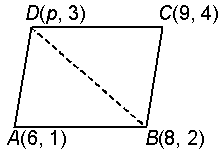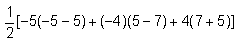# CBSE 10th Mathematics | Coordinate Geometry | Area of A Triangle

## Coordinate Geometry | Area of A Triangle

The area of a DABC with vertices$\displaystyle A\left( {{x}_{1}},{{y}_{1}} \right),B\left( {{x}_{2}},{{y}_{2}} \right)$ and$\displaystyle C\left( {{x}_{3}},{{y}_{3}} \right)$ is given by area$\displaystyle \left( \Delta ABC \right)=\left| \frac{1}{2}\left\{ {{x}_{1}}\left( {{y}_{2}}-{{y}_{3}} \right)+{{x}_{2}}\left( {{y}_{3}}-{{y}_{1}} \right)+{{x}_{3}}\left( {{y}_{1}}-{{y}_{2}} \right) \right\} \right|.$

Proof :Let$\displaystyle A\left( {{x}_{1}},{{y}_{1}} \right),B\left( {{x}_{2}},{{y}_{2}} \right)$ and$\displaystyle C\left( {{x}_{3}},{{y}_{3}} \right)$ be the vertices of the given DABC.

Draw AP, BM and CN perpendiculars to the x-axis.

Then,$\displaystyle MP=\left( {{x}_{1}}-{{x}_{2}} \right),PN\left( {{x}_{3}}-{{x}_{1}} \right)$

and MN =$\displaystyle \left( {{x}_{3}}-{{x}_{2}} \right)$

\     area of DABC

= ar (trap. BMPA) + ar (trap.. APNC) ar (trap. BMNC)

=$\displaystyle \left\{ \frac{1}{2}\left( AP+BM \right)\times MP \right\}+\left\{ \frac{1}{2}\left( AP+CN \right)\times PN \right\}-\left\{ \frac{1}{2}\left( BM+CN \right)\times MN \right\}$

=$\displaystyle \frac{1}{2}\left( {{y}_{1}}+{{y}_{2}} \right)\left( {{x}_{1}}-{{x}_{2}} \right)+\frac{1}{2}\left( {{y}_{1}}+{{y}_{3}} \right)\left( {{x}_{3}}-{{x}_{1}} \right)-\frac{1}{2}\left( {{y}_{2}}+{{y}_{3}} \right)\left( {{x}_{3}}-{{x}_{2}} \right)$

=$\displaystyle \frac{1}{2}\left[ {{x}_{1}}\left( {{y}_{1}}+{{y}_{2}}-{{y}_{1}}-{{y}_{3}} \right)+{{x}_{2}}\left( {{y}_{2}}+{{y}_{3}}-{{y}_{1}}-{{y}_{2}} \right)+{{x}_{3}}\left( {{y}_{1}}+{{y}_{3}}-{{y}_{2}}-{{y}_{3}} \right) \right]$$\displaystyle =\frac{1}{2}\left\{ {{x}_{1}}\left( {{y}_{2}}-{{y}_{3}} \right)+{{x}_{2}}\left( {{y}_{3}}-{{y}_{1}} \right)+{{x}_{3}}\left( {{y}_{1}}-{{y}_{2}} \right) \right\}.$

Since the area is never negative, we have

area$\displaystyle \left( \Delta ABC \right)=\frac{1}{2}\left| \left\{ {{x}_{1}}\left( {{y}_{2}}-{{y}_{3}} \right)+{{x}_{2}}\left( {{y}_{3}}-{{y}_{1}} \right)+{{x}_{3}}\left( {{y}_{1}}-{{y}_{2}} \right) \right\} \right|$

Condition for Collinearity of Three Points

Let the given points be$\displaystyle A\left( {{x}_{1}},{{y}_{1}} \right),B\left( {{x}_{2}},{{y}_{2}} \right)$ and$\displaystyle \left( {{x}_{3}},{{y}_{3}} \right)$.

Then, A, B and C are collinear

Þ area of DABC = 0

Þ$\displaystyle \frac{1}{2}.\left\{ {{x}_{1}}\left( {{y}_{2}}-{{y}_{3}} \right)+{{x}_{2}}\left( {{y}_{3}}-{{y}_{1}} \right)+{{x}_{3}}\left( {{y}_{1}}-{{y}_{2}} \right) \right\}=0$

Þ$\displaystyle {{x}_{1}}\left( {{y}_{2}}-{{y}_{3}} \right)+{{x}_{2}}\left( {{y}_{3}}-{{y}_{1}} \right)+{{x}_{3}}\left( {{y}_{1}}-{{y}_{2}} \right)=0$

### Solution:

The area of the triangle formed by the vertices A(1, –1), B(–4, 6) and C(–3, –5) is given by$\displaystyle \frac{1}{2}[1(6+5)+(-4)(-5+1)+(-3)(-1-6)]$ =$\displaystyle \frac{1}{2}(11+16+21)=24$

So, the area of the triangle is 24 square units.

### Solution:

Since the given points are collinear, the area of the triangle will be 0,

Þ$\displaystyle \frac{1}{2}[2(k+3)+4(-3-3)+6(3-k)]=0$

Þ$\displaystyle \frac{1}{2}[2k+6-24+18-6k]=0$

Þ$\displaystyle \frac{1}{2}(-4k)=0$

Therefore, k = 0

### Solution:By joining B to D, two triangles ABD and BCD are formed.

Now the area of DABD

==$\displaystyle \frac{1}{2}(50+8+48)=\frac{106}{2}=53$ square units

Also, the area of DBCD

=$\displaystyle \frac{1}{2}[-4(-6-5)-1(5+5)+4(-5+6)]$

=$\displaystyle \frac{1}{2}(44-10+4)=19$ square units

So, the area of quadrilateral ABCD = 53 + 19 = 72 square units.

### Solution:

Let D be the area of the triangle formed by the points (a, b + c), (b, c + a) and (c, a + b)

Þ$\displaystyle \Delta =\frac{1}{2}\left| \left\{ a(c+a)+b(a+b)+c(b+c) \right\}-\left\{ b(b+c)+c(c+a)+a(a+b) \right\} \right|$

Þ$\displaystyle \Delta =\frac{1}{2}\left| (ac+{{a}^{2}}+ab+{{b}^{2}}+bc+{{c}^{2}})-({{b}^{2}}+bc+{{c}^{2}}+ca+{{a}^{2}}+ab) \right|$

Þ    D = 0

Since area is zero \ the given points are collinear.

### Solution:

The vertices of the given DABC are$\displaystyle A\left( k,2k \right),B\left( -2,6 \right)$ and$\displaystyle C\left( 3,1 \right)$.

\$\displaystyle {{x}_{1}}=k,\,{{y}_{1}}=2k,{{x}_{2}}=-2,{{y}_{2}}=6$ and$\displaystyle {{x}_{3}}=3,{{y}_{3}}=1$

Area of$\displaystyle \Delta ABC=\frac{1}{2}\left| \left\{ {{x}_{1}}\left( {{y}_{2}}-{{y}_{3}} \right)+{{x}_{2}}\left( {{y}_{3}}-{{y}_{1}} \right)+{{x}_{3}}\left( {{y}_{1}}-{{y}_{2}} \right) \right\} \right|$

=$\displaystyle \frac{1}{2}\left| k\left( 6-1 \right)-2\left( 1-2k \right)+3\left( 2k-6 \right) \right|$

=$\displaystyle \frac{1}{2}\left| 5k-2+4k+6k-18 \right|$

=$\displaystyle \frac{1}{2}\left| 15k-20 \right|$ sq units.

But, area of DABC = 5 sq units     [given]

\$\displaystyle \frac{1}{2}\left| 15k-20 \right|=5\,\,\,\,\,\,\Rightarrow \,\,\,\,\,\left| 15k-20 \right|=10$

Þ$\displaystyle \left( 15k-20 \right)=10$ or$\displaystyle \left( 15k-20 \right)=-10$

Þ$\displaystyle k=2$ or$\displaystyle k=\frac{2}{3}$.

Hence,$\displaystyle k=2$ or$\displaystyle k=\frac{2}{3}$

This site uses Akismet to reduce spam. Learn how your comment data is processed.